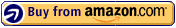# Grade 4 Geometry & Measurement

Your child will progress gradually through our activities and master area, volume, circumference, and diameter. Children learn to measure angles with a protractor and calculate decimal and fraction problems with ease.

-Read and write fractions on a number line
-Read and write decimals on a number line
-Understand and apply concepts of lines, angles, and circles
-Draw two-dimensional figures
-Measure and find the area and perimeter of rectangular shapes using appropriate units.
-Measure volume using standard cubic units.
-Measure surface area of cubes and rectangular prisms by adding up the area of the faces
-Calculate elapsed time in hours and half hours
-Calculate elapsed time in days and weeks

8 1/2 x 11 inches. paperback. 96 pages. full color.

ISBN: 1-934968-67-6 ISBN: 978-1-934968-67-3

#### US: \$7.95 | CA: \$8.95